# System Modeling

In order to develop accurate control systems for the quadrotor platform, it is necessary to develop and analyze the equations of motion that define the quadrotor system. The quadrotor is defined by a set of non-linear equations which make accurate simulation as well as control difficult. Once all the components of the equations are developed, it is possible to simplify the equations of motion after making several assumptions about the method which the quadrotor will be operated. The goal is to make a model that is simple while still being as realistic as possible.

###### Variable and Coordinate System Definition

The quadrotor vehicle operates on the concept of variable torques and thrusts. Each rotor consists of a brushless DC motor and rotor with a fixed pitch. The motors are arranged in pairs along the horizontal and vertical axes with the forward pair rotating clockwise and the horizontal pair rotating counter-clockwise. This design results in the reaction torques from the pairs of motors being exactly opposed by each other if they are all spinning at the same speed. The elimination of the rotating moment allows the vehicle to maintain a constant heading while hovering. Yaw is controlled by varying the speeds of the pairs of motors to create a non-zero net counter torque. Altitude is controlled by varying the thrust from each motor by equal amounts to provide a net thrust vector and without a rotational moment. To move in the lateral directions, the relative speeds of each motor in the lateral pair are varied to create a desired lateral thrust offset. The simple design results in small platforms which are battery operated, able to perform a stable hover, and safe to use in indoor environments. The quadrotor is classified as an under-actuated system. While the quadrotor can move in 6 degrees of freedom (3 translational and 3 rotational), there are only 4 inputs that can be controlled (the speeds of the 4 motors). As will be shown below, the rotational and translational dynamics are coupled which presents an interesting control problem.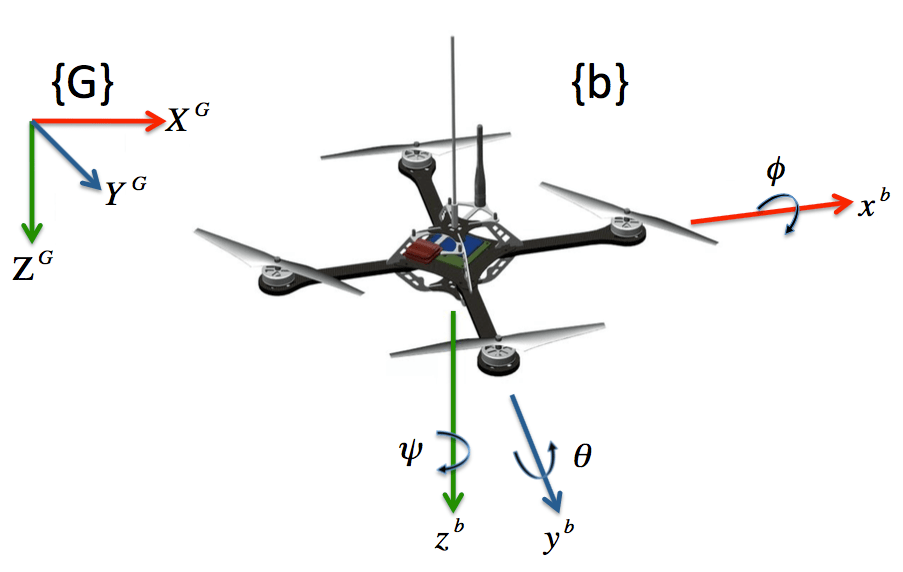The basic quadrotor structure used for the model development is shown in the figure above depicting the Euler angles of roll, pitch, and yaw, a body coordinate frame {b}, and the global coordinate frame {G}. This model relies on several assumptions:

• The quadrotor structure is rigid and symmetrical with a center of mass aligned with the center of the body frame of the vehicle.
• The thrust and drag of each motor is proportional to the square of the motor velocity.
• The propellers are considered to be rigid and therefore blade flapping is negligible (deformation of propeller blades due to high velocities and flexible material).
• The Earth is flat and non-rotating (difference of gravity by altitude or the spin of the earth is negligible)
• Surrounding fluid velocities (wind) are negligible.
• Ground effect is negligible.

The complete list of state variables is shown below. This notation uses notation taken from the aeronautics literature, specifically the North, East, Down (NED) coordinate system. The position of the quadrotor is given in the global frame while the velocity and angular velocity are defined in the quadrotor body frame. It is important to note that different common sensors produce measurements in both coordinate systems. GPS, for example, measures the quadrotor’s position and ground speed in the global frame while accelerometers and rate gyros produce measurements in the body frame. For more advanced navigation including waypoint trajectory following, the waypoints are given in the global coordinate system.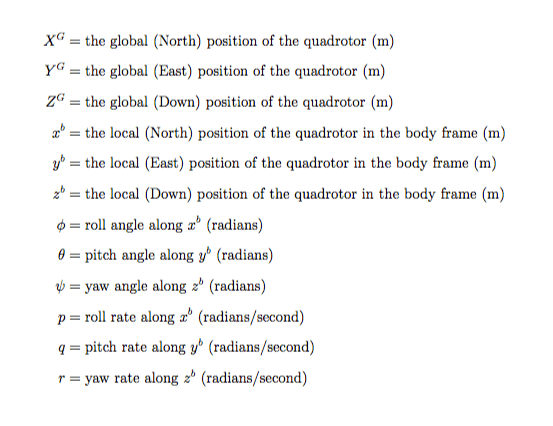###### Translational Kinematics

The state variables for velocity are in the body frame but the state variables for position are in the global frame. Therefore, it is necessary to define a rotation matrix to transform variables between the coordinate systems. The transformation between the global and body coordinate frames is described below.###### Rotational Kinematics

Since the angular rates are defined in the body frame and the Euler angles are defined in intermediate coordinate frames, we can use the rotation matrix derived above to determine the relationship between the angular rates and the time derivatives of the Euler angles as shown below. The angular velocities are vectors pointing along each axis of rotation and are not equal to the time derivative of the Euler angles. The derivation below assumes that the time derivative of each Euler rate is small. See this page for more detailed information about Euler angles.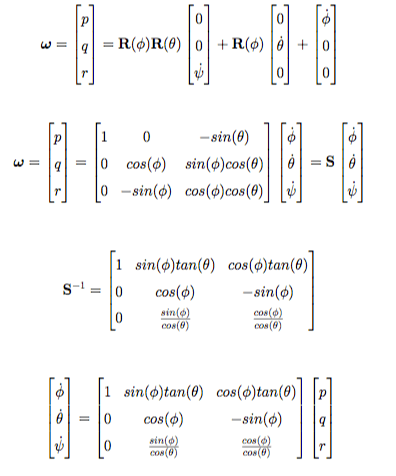If the Euler angles are assumed to be small (near 0), then the S matrix becomes the identity matrix and the angular rates are roughly equal to the time derivative of the Euler angles.

###### Brushless Motor Equations of Motion

Quadrotors use varying types of brushless motors to generate the thrust and torques needed to control the platform. Each motor is powered by electric batteries carries on-board the quadrotor platform. Electronic Speed Controllers (ESCs) receive the desired motor speed output by the flight controller and send those commands to each individual motor. A basic free body diagram is shown which depicts the thrusts and forces of each motor on the quadrotor. Note that the z axis has been defined negative in the direction of gravity and the opposite direction of the motor force vectors.The first series of equations below derive the torque and power equations of a typical brushless motor. We assume that the motor resistance and the current with no load are approximately 0. Since all the motors are the same on the quadrotor, a single model applies to every motor. We derive the equation for the amount of power a motor produces in a single simplified equation.The following equations use the power and torque equations derived above to develop a generalized thrust equation. The consumed power at hover is the ideal power required to generate thrust by moving a column of air. We assume vehicle speeds are low, so the velocity at hover is the velocity of the air when hovering. From this equation, it is evident that larger propellers result in less power consumed. While each rotor leaves behind a wake as it moves, we assume this free stream air velocity is zero. This results in the thrust of each motor being proportional to the product of the square of the angular velocity of the rotor shaft and a lift coefficient. This equation depicts the relationship between motor speed and resultant force of thrust, which is the basis for stable control of the vehicle.###### Translational Dynamics

The linear equations of motion are defined in the global reference frame. The acceleration of the quadrotor in the global frame is equal to the sum of force of gravity, the thrust force of the motors, and linear friction force resulting in drag. The thrust vector in the body frame is transformed into the global frame using the rotation matrix defined previously. The resultant force generated by the four motors in the global coordinate frame is defined below. Additionally, the resultant global drag force along each translational axis is defined below as the product of drag coefficients and linear velocities in each direction in the global frame. This is a simple model of fluid friction. More complex modeling would involve separate friction constants for each axis as well as modeling the friction in the body frame instead of the inertial frame.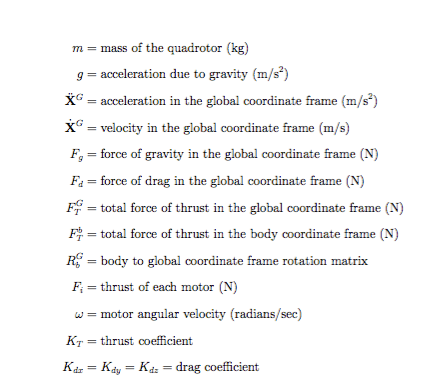###### Rotational Dynamics

The rotational equations of motion are defined in the body frame so that the rotations can be computed about the quadrotor’s center and not the center of the global coordinate frame. Since we assumed the quadrotor to be symmetrical, the quadrotor moment of inertia matrix is symmetrical. These equations are comprised of three terms including aerodynamic effects and motor torques. First, the gyroscopic effect resulting from the rigid body rotation. Second, the motors produce a rolling, pitching, and yawing torque. The torque due to drag is shown to be proportional to the product of a drag constant and the square of the rotor’s angular velocity. This drag equation assumes that the quadrotor is operating in stable flight and that the propellers are maintaining a constant thrust and not accelerating. This assumption results in the torque about the global z axis being equal to the torque due to drag. Third, the cross product which describes the gyroscopic effect resulting from the propeller rotation coupled with the body rotation. This is due to the fact that the rotor’s axis of rotation is itself moving with the angular velocity of the frame.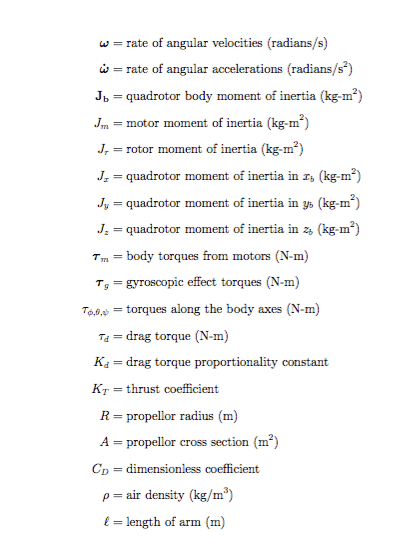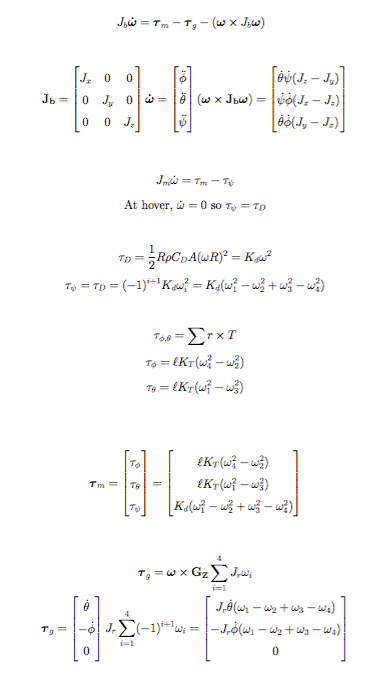###### Equations of Motion

Solving for the quadrotor’s global translational acceleration and body frame angular accelerations leads to the following equations. We use the global frame for translational position since that is the same coordinate frame as our GPS sensor. Similarly, the body frame is chosen for attitude since the IMU (accelerometer, magnetometer, and gyroscope) also make measurements in the body frame. These equations are both non-linear and highly coupled. They can be simplified using reasonable assumptions in order to design and implement stable control systems.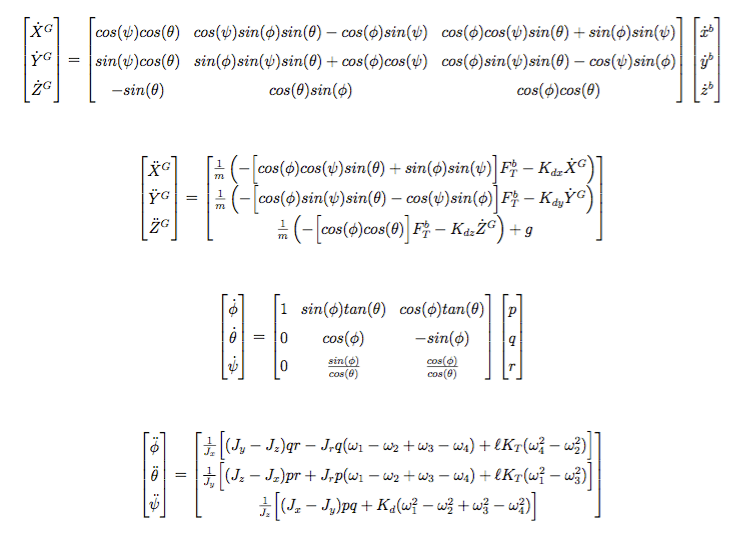###### Resources:

Coordinate Systems:

CH Robotics, “Understanding Euler Angles

Grewal, Mohinder S., Lawrence R. Weill, and Angus P. Andrews. Global positioning systems, inertial navigation, and integration. John Wiley & Sons, 2007.

Beard, Randal W. “Quadrotor dynamics and control.” Brigham Young University (2008).

Bouadi, H., M. Bouchoucha, and M. Tadjine. “Sliding mode control based on backstepping approach for an UAV type-quadrotor.” World Academy of Science, Engineering and Technology 26 (2007): 22-27.

Cai, Guowei, Ben M. Chen, and Tong H. Lee. Unmanned Rotorcraft Systems. New York: Springer, 2011

Carrillo, L. R. G., López, A. E. D., Lozano, R. and Pégard, C. (2013). Quad Rotorcraft Control, Advances in Industrial Control, Springer-Verlag, London.

DiCesare, Antonio. Design Optimization of a Quad-Rotor Capable of Autonomous Flight. Diss. WORCESTER POLYTECHNIC INSTITUTE, 2008.

Gibiansky, Andrew. Quadcopter Dynamics and Simulation.

Hoffmann, Gabriel M. Autonomy for sensor-rich vehicles: Interaction between sensing and control actions. ProQuest, 2008.

Koks, Don and Defence Science and Technology Organisation (Australia). Systems Sciences Laboratory.  Using rotations to build aerospace coordinate systems. Edinburgh, S. Aust  2005

Mian, Ashfaq Ahmad, and Dao-bo Wang. “Dynamic modeling and nonlinear control strategy for an underactuated quad rotor rotorcraft.” Journal of Zhejiang University Science A 9.4 (2008): 539-545.

Min, Byung-Cheol, et al. “Development of a micro quad-rotor UAV for monitoring an Indoor environment.” Advances in Robotics. Springer Berlin Heidelberg, 2009. 262-271.

R. N. Jazar, Theory of Applied Robotics: Kinematics, Dynamics, and Control, Springer, New York, NY, USA, 2nd edition, 2010.

Tayebi, A.; McGilvray, S., “Attitude stabilization of a four-rotor aerial robot,” Decision and Control, 2004. CDC. 43rd IEEE Conference on , vol.2, no., pp.1216,1221 Vol.2, 14-17 Dec. 2004

Tayebi, Abdelhamid, and Stephen McGilvray. “Attitude stabilization of a VTOL quadrotor aircraft.” Control Systems Technology, IEEE Transactions on 14.3 (2006): 562-571.

[simple-social-share]

## 2 thoughts on “System Modeling”

1.vasanthakumar v says:

Here how to find the values of P, Q, R, Can it be from psidouble dot, phidoubledot,thetadoubledot.

2.wilselby says:

Thanks! Do you have the specific error?